Computers, Materials & ContinuaDOI:10.32604/cmc.2022.017698Article

Algorithmic Scheme for Concurrent Detection and Classification of Printed Circuit Board Defects

Faculty of Engineering, Rajamangala University of Technology Thanyaburi (Main Campus), Khlong Luang, Pathum Thani, 12110, Thailand
*Corresponding Author: Jakkree Srinonchat. Email: jakkree.s@en.rmutt.ac.th
Received: 07 February 2021; Accepted: 31 August 2021

Abstract: An ideal printed circuit board (PCB) defect inspection system can detect defects and classify PCB defect types. Existing defect inspection technologies can identify defects but fail to classify all PCB defect types. This research thus proposes an algorithmic scheme that can detect and categorize all 14-known PCB defect types. In the proposed algorithmic scheme, fuzzy c-means clustering is used for image segmentation via image subtraction prior to defect detection. Arithmetic and logic operations, the circle hough transform (CHT), morphological reconstruction (MR), and connected component labeling (CCL) are used in defect classification. The algorithmic scheme achieves 100% defect detection and 99.05% defect classification accuracies. The novelty of this research lies in the concurrent use of CHT, MR, and CCL algorithms to accurately detect and classify all 14-known PCB defect types and determine the defect characteristics such as the location, area, and nature of defects. This information is helpful in electronic parts manufacturing for finding the root causes of PCB defects and appropriately adjusting the manufacturing process. Moreover, the algorithmic scheme can be integrated into machine vision to streamline the manufacturing process, improve the PCB quality, and lower the production cost.

Keywords: : PCB inspection; PCB defect types; defect detection; defect classification; image processing

1  Introduction

In electronic component manufacturing, a system for defect inspection of printed circuit boards (PCB) prevents defective PCBs from advancing to the subsequent stage. Because of higher efficiency, accuracy, and productivity compared to human inspectors, PCB defect inspection technologies based on automatic image-processing techniques have been increasingly deployed.

There are three PCB defect inspection approaches: referential, non-referential, and hybrid . PCB defects are detected in the referential approach by comparing a PCB image against a template PCB image. At the same time, in the non-referential method, a pre-defined mathematical rule is established to detect PCB defects. As for the hybrid approach, it uses a combination of referential and non-referential methods. However, the mentioned PCB defect inspection approaches mainly concentrate on defect detection, which cannot identify the root causes of defects. Therefore, researchers need to work in both PCB defect detection and PCB defect classification simultaneously.

In PCB fabrication, there are 14-known PCB defect types: Breakout, Pin hole, Open circuit, Under etch, Mouse bite, Missing conductor, Spur, Short circuit, Wrong size hole, Conductor too close, Spurious copper, Excessive short, Missing hole, and Over etch . PCB defects consist of two groups: functional and cosmetic defects . Functional defects cause the PCB to malfunction, whereas cosmetic defects affect the PCB appearance and might impair the performance of the PCB. ‏ Fig. 1 illustrates a template PCB and a defective PCB containing 14-known defect types. In , the 14-known PCB defects are further categorized by processes (i.e., drilling and etching) and characteristics (i.e., missing copper and excess copper), as shown in ‏ Tab. 1.

In , image subtraction is used to detect PCB defects, and arithmetic operations are utilized to classify defects. Meanwhile, in , image subtraction and morphological operations are applied to detect and classify PCB defects. However, these techniques can classify only four to eight defect types (out of 14-known defect types). Convolutional neural network (CNN) methods are employed to classify PCB defects in , but their classification capabilities are low. In , all 14-known defect types are initially categorized into five classes, and a classification algorithm is applied to classify defect types. However, the algorithm can only separate the defects into five categories and cannot satisfy 14 types. Moreover, the work of  is then improved by applying a segmentation exercise, which is described in . These works can only expand five classes to seven classes, and in , the number is improved to 11 categories. However, they still cannot identify all 14 defect types. Since each type of PCB defect occurs due to different causes and processes, the classification of 14 defect types can help determine the cause of the error and therefore reduce long-term production costs.

An image segmentation algorithm based on mathematical and morphological tools is proposed to divide individual PCB images into four segments: hole, pad, thick line, and thin line. The algorithm is usually employed as a pre-processing step for classification tasks . In , a k-nearest neighbor algorithm is utilized to classify the defects, and the algorithm can only classify all 14-known defect types with 70%–80% accuracy. Nonetheless, the high-accuracy defect classification procedure is essential for an inspection system during PCB production processes.

Accordingly, this study proposes an algorithmic scheme to detect and classify all 14-known PCB defect types with at least 90% accuracy. In the proposed defect inspection system, a fuzzy c-means clustering algorithm is used in image segmentation. Image subtraction is utilized for defect detection, and the circle Hough transform (CHT), morphological reconstruction (MR), and connected component labelling (CCL) algorithms are employed in defect classification.

2  The Algorithmic Scheme for PCB Defect Detection and Classification

In this research, the PCB detection and classification system uses the referential approach based on 14-known PCB defect types. The proposed system consists of four steps: pre-processing, image segmentation (Section 2.‏1), defect detection, and defect classification (Section 2.‏2).

Pre-Processing and Image Segmentation

Fig. 2 illustrates the flowchart of the pre-processing and image segmentation processes. During the pre-processing stage, RGB color images (containing PCB defects) are converted into grayscale images and median-filtered to remove the noise from images. An affine transform is used to scale, rotate, and translate the median-filtered images before image segmentation using fuzzy c-means clustering (i.e., the constituent images of an input image).Figure 1: Template and defective PCBs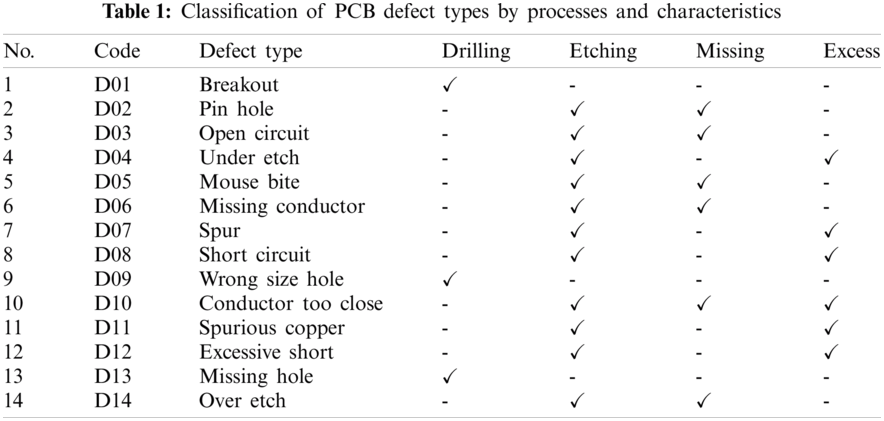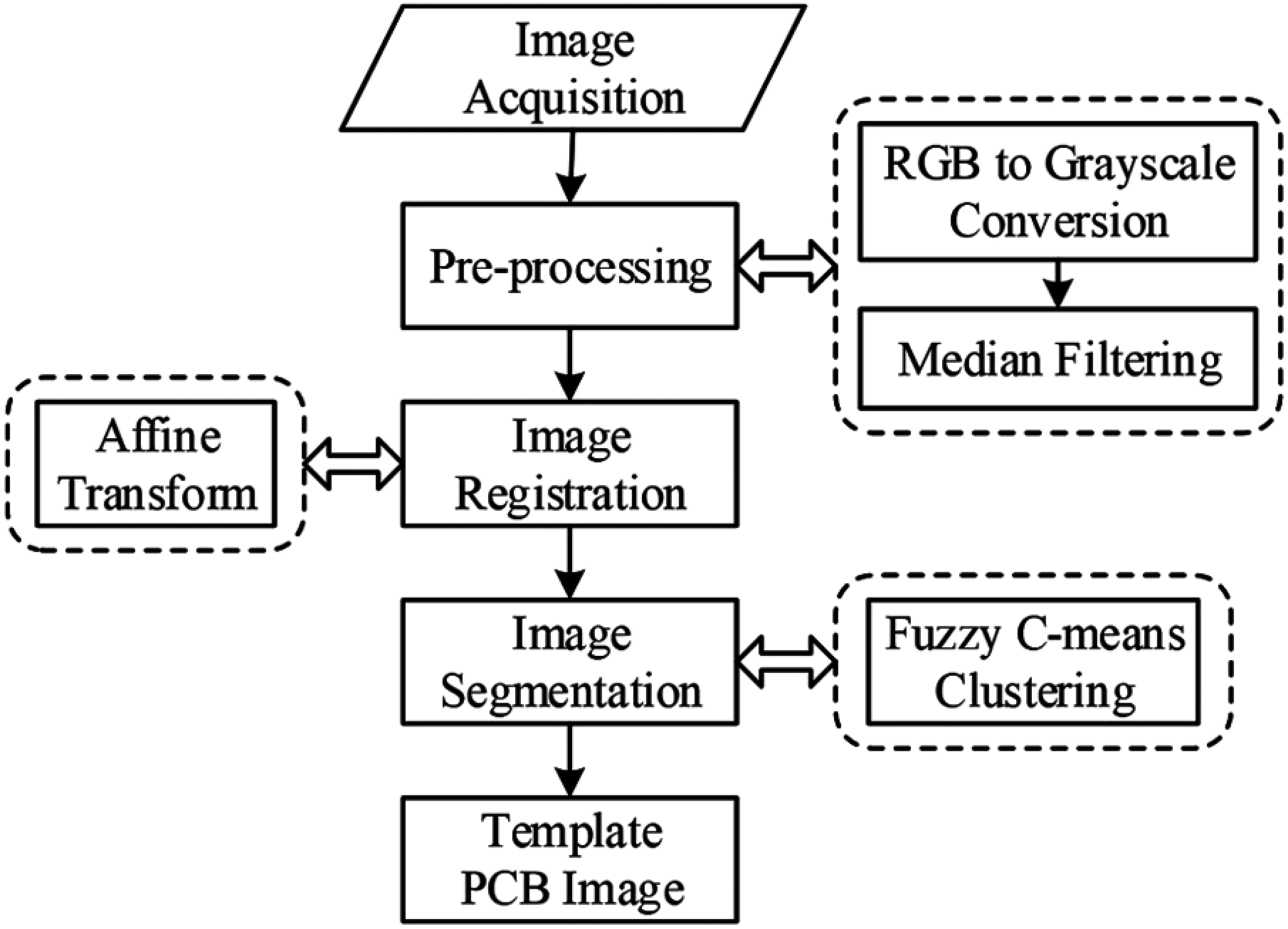Figure 2: The flowchart of pre-processing and image segmentation

The RGB color images are converted into grayscale images by individual pixels following Eq. (1), where R, G, and B represent red, green, and blue channels in the color image, respectively:

Grayscale=0.299R+0.587G+0.114B. (1)

Then, median filtering is used to remove the noise from images, whereby the median grayscale is assigned to individual pixels in the image . The median greyscale is calculated by Eq. (2) as follows:

g(x,y)=median{f(m,n),(m,nWxy)}, (2)

where f(m,n) is the initial grayscale, g(x,y) is the median greyscale, and W is the 3 × 3 kernel, of which m and n are the number of columns and rows, respectively.

Furthermore, the affine transform algorithm is used to scale, rotate, and transform the median-filtered images. The affine transform algorithm is mathematically expressed in Eq. (3), consisting of six degrees of freedom: tx and ty for transformation, a11 and a12 for scaling, and a21and a22 for rotation :

f(x^,y^,t1)=f(a11x+a12y+tx,a21x+a22y+ty,t), (3)

where f(x^,y^,t1) and f(x,y,t) are the affine-transform and the median-filtered images, respectively.

Next, in image segmentation, the fuzzy c-means clustering algorithm is utilized to separate circuit parts, including holes, pads, and traces from the background. The algorithm uses the nearest distance between any given pixel and its cluster centroid using the Euclidean distance as described in Eqs. (4)–(6) :

J=j=1ki=1nuijm||xi(j)cj2||, (4)

uij=1q=1k(||xicj||||xicq||)2m1, (5)

cj=i=1n(xiuijm)i=1n(uijm), (6)

where J is the nearest distance between a given pixel and its cluster centroid, uij is a data member of the cluster center, cj is the centroid of the cluster j, k is the number of clusters, n is the total number of data, and xi(j) is the data at pixel i and cluster j.

In addition, the fuzzy c-means clustering algorithm divides each median-filtered image (i.e., input image) into 32 rectangular-shaped segmental images. The segmental images are subsequently designated into clusters according to standard features and characteristics. The constituent images (or segmental images) belonging to various clusters then undergo defect detection and classification.

In this study, 500 input images contain various degrees of 14-known PCB defects. In segmentation, each input image is divided into 32 segmental images using fuzzy c-means clustering, generating 16,000 segmental images. The segmental images are individually assigned to clusters according to common characteristics, resulting in 100 clusters. For verification purposes, this study utilizes 10,000 segmental images for testing the defect detection and classification algorithm.

Defect Detection and Classification

Fig. 3 illustrates the flowchart of detection and classification of 14-known PCB defects. In defect detection, image subtraction is utilized to compare the segmental images against the reference image (i.e., the defect-free segmental image). A negative value (n(x,y)) indicates excess copper (Eq. (7)) and a positive value (p(x,y)) means missing copper (Eq. (8)). A negative or positive subtraction value stands for defective PCB, whereas a zero-subtraction value signifies non-defective PCB, as follows:

n(x,y)=g(x,y)f(x,y), (7)

p(x,y)=f(x,y)g(x,y), (8)

where g(x,y) is the reference PCB image, and f(x,y) is the defect-containing segmental PCB image.

The PCB defect types with a positive subtraction value include Breakout, Pin hole, Open circuit, Mouse bite, Missing conductor, and Over etch. In contrast, those with a negative subtraction value contain Under etch, Spur, Short circuit, Wrong size hole, Spurious copper, Excessive short, and Missing hole. The Conductor too close has either a positive or negative subtraction value, thereby presenting classification challenges.

CHT, MR, and CCL algorithms are utilized along with image subtraction to enhance defect detection. Specifically, the CHT algorithm detects and classifies circular patterns by transforming a set of feature points in the image space into a set of accumulated votes in parameter space. The votes at each feature point are accumulated in an accumulator array for all parameter combinations. The highest number of votes in the array elements indicates the presence of a circular pattern. A circle is described in Eq. (9), and its parameters are defined in Eqs. (10) and (11) . The hole pad pattern of the defect-containing segmental images (hp(x,y)) is determined in Eqs. (9)–(11):

(xx0)2+(yy0)2=r2, (9)

x=x0+rcos(θ), (10)

y=y0+rsin(θ), (11)

where x0 and y0 are the center coordinates, r is the circle radius, and θ is the rotational angle of the segmental image (0–360°).Figure 3: The flowchart of defect detection and classification

The MR algorithm sequentially classifies segmental images by features (i.e., holes, pads, and traces) and then transforms the connected background pixels into foreground pixels until the object boundaries are reached. The MR algorithm for flood-filling is expressed in Eq. (12), where f(x,y) denotes the segmental image and fm(x,y) is the flood-fill segmental image :

fm(x,y)={1f(x,y),if(x,y) on border of f(x,y)0Otherwise (12)

The CCL algorithm scans segmental images, groups pixels into components by pixel connectivity, and assigns labels to individual pixels until the boundaries are reached . In this research, binary CCL (i.e., 0 and 1) combines the connected copper traces into one group. The number of groups and sizes of the groups are used to classify defect types.

The Algorithmic Scheme of Defect Detection and Defect Classification

The inputs of the algorithmic scheme are f(x,y), g(x,y), n(x,y), p(x,y), hp(x,y), and fm(x,y), and the outputs are the 14 known PCB defects (denoted as D01-D14). The experiment use the computer hardware with an Intel i5-8250U CPU @ 1.60 GHz and 8 GB RAM. The sequential steps of the algorithmic scheme are as follows:

Step 1: Find excess copper in hole pads using (n(x,y) & hp(x,y)).

Step 2: Apply the CCL algorithm to validate, and CHT classifies the defect as either a Wrong size hole (D09) or a Missing hole (D13).

Step 3: Find missing copper in hole pads using (p(x,y) & hp(x,y)).

Step 4: Apply the CCL algorithm to validate, and CHT classifies the defect as Breakout (D01).

Step 5: Find Pin hole defect (D02) from D01  (p(x,y) & (g(x,y) fm(x,y))).

Step 6: Or else, the defect is an Excessive short (D12).

Step 7: Apply the CCL algorithm to determine the number and size of groups.

Step 8: Add negative pixels to the defect-containing segmental image and re-apply the CCL algorithm to determine the number and size of groups.

Step 9: Comparing Steps 7 and 8. If the number and size of groups decrease, the defect is a Short circuit defect (D08). Otherwise, the defect is a Spurious copper defect (D11).

Step 10: Add positive pixels to the defect-containing segmental image and re-apply the CCL algorithm to determine the number and size of groups.

Step 11: Comparing Steps 7 and 10. If the number of groups increases and the group size is smaller than the square of the trace width, the defect is an Open circuit defect (D03). If the number of groups increases and the size of the group is larger than the square of the trace width, it is a Missing conductor defect (D06).

Step 12: If the number of groups remains unchanged, proceed to steps 13–17.

Step 13: If the defect has negative pixels and is asymmetric, it is a Spur defect (D07).

Step 14: If the defect has positive pixels and is asymmetric, it is a Mouse bite defect (D05).

Step 15: If the defect has either negative or positive pixels and is symmetric, it is a Conductor too close defect (D10).

Step 16: If the defect has negative pixels and is symmetric, it is an Under etch defect (D04).

Step 17: If the defect has positive pixels and is symmetric, it is an Over etch defect (D14).

Step 18: If the defect does not fall into any of the 14 categories, it is another defect (D00).

Step 19: About Steps 1–18, all 14-known PCB defect types are detected and classified.

3  Experimental Results and Discussion

PCB Input and Segmental Images

As previously stated, 500 input images contain various degrees of 14-known PCB defects. The input images are segmented using the fuzzy c-means clustering algorithm. In the segmentation, each input image is divided into 32 segmental images, resulting in 16,000 segmental images. The segmental images are individually assigned to one of 100 clusters according to their common characteristics. In the meanwhile, this study uses 10,000 segmental images to test the algorithmic scheme for defect detection and classification. Fig. 4 depicts an input image and corresponding defect-containing segmental images.

Image Segmentation Performance

This study utilizes fuzzy c-means clustering in image segmentation. Before that, simulations are carried out to determine the optimal algorithmic scheme among four alternative image segmentation algorithms, i.e., global thresholding, adaptive thresholding, k-means clustering, and fuzzy c-means clustering. Besides, the performance metrics include intersection over union (IoU), accuracy, precision, recall, and elapse time. The IoU, accuracy, precision, and recall are calculated by Eqs. (13)(16) . Fig. 5 compares the performance (mean values) of four alternative image segmentation algorithms, and Tab. 2 tabulates their respective standard deviations (SD). The SD is calculated following Eq. (17).

IoU=GroundThruthPredictionGroundThruthPrediction, (13)

Accuracy=(TP)+(TN)(TP)+(FP)+(TN)+(FN), (14)

Precision=(TP)(TP)+(FP), (15)

Recall=(TP)(TP)+(FN), (16)

where TP, TN, FP, and FN are the number of true positives, true negatives, false positives, and false negatives, respectively.

SD=1Ni=1N(xix¯)2, (17)

where xi is a value in the data set, x¯ is the mean of the data set, and N is the number of data points in the population.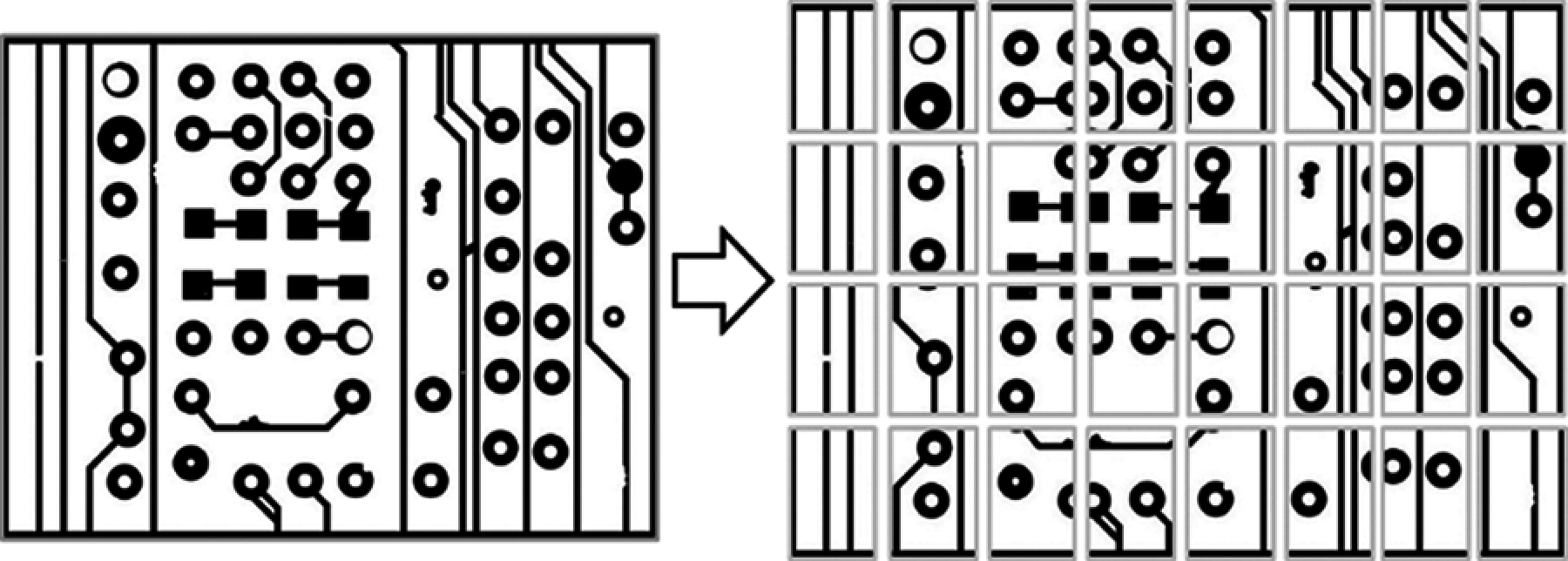Figure 4: An example of an input image and the corresponding segmental images

In Fig. 5, fuzzy c-means clustering achieves the highest precision (99.34%), accuracy (98.62%), recall (98.17%), and IoU (0.9574). Nonetheless, the elapsed time of fuzzy c-means clustering is longer than the other segmentation algorithms, which can be overcome by upgrading the computer processing unit. As shown in Tab. 2, the standard deviations are relatively small, suggesting minimal deviation from the means.

Defect Detection Performance

The image subtraction algorithm is utilized to detect defects on PCB by comparing the segmental images of input images against the reference image (i.e., defect-free segmental image). A negative or positive subtraction value indicates a defective PCB, whereas a zero-subtraction value signifies a non-defective PCB.

The PCB defect types with a positive subtraction value include Breakout, Pin hole, Open circuit, Mouse bite, Missing conductor, and Over etch. In contrast, those with a negative subtraction value include Under etch, Spur, Short circuit, Wrong size hole, Spurious copper, Excessive short, and Missing hole. The Conductor too close defect has either a positive or negative subtraction value. Figs. 6a6c illustrate an example of defect detection results using image subtraction, with the positive values (as represented by white-colored marks) indicating detected defects. The image subtraction algorithm can achieve 100% defect detection accuracy.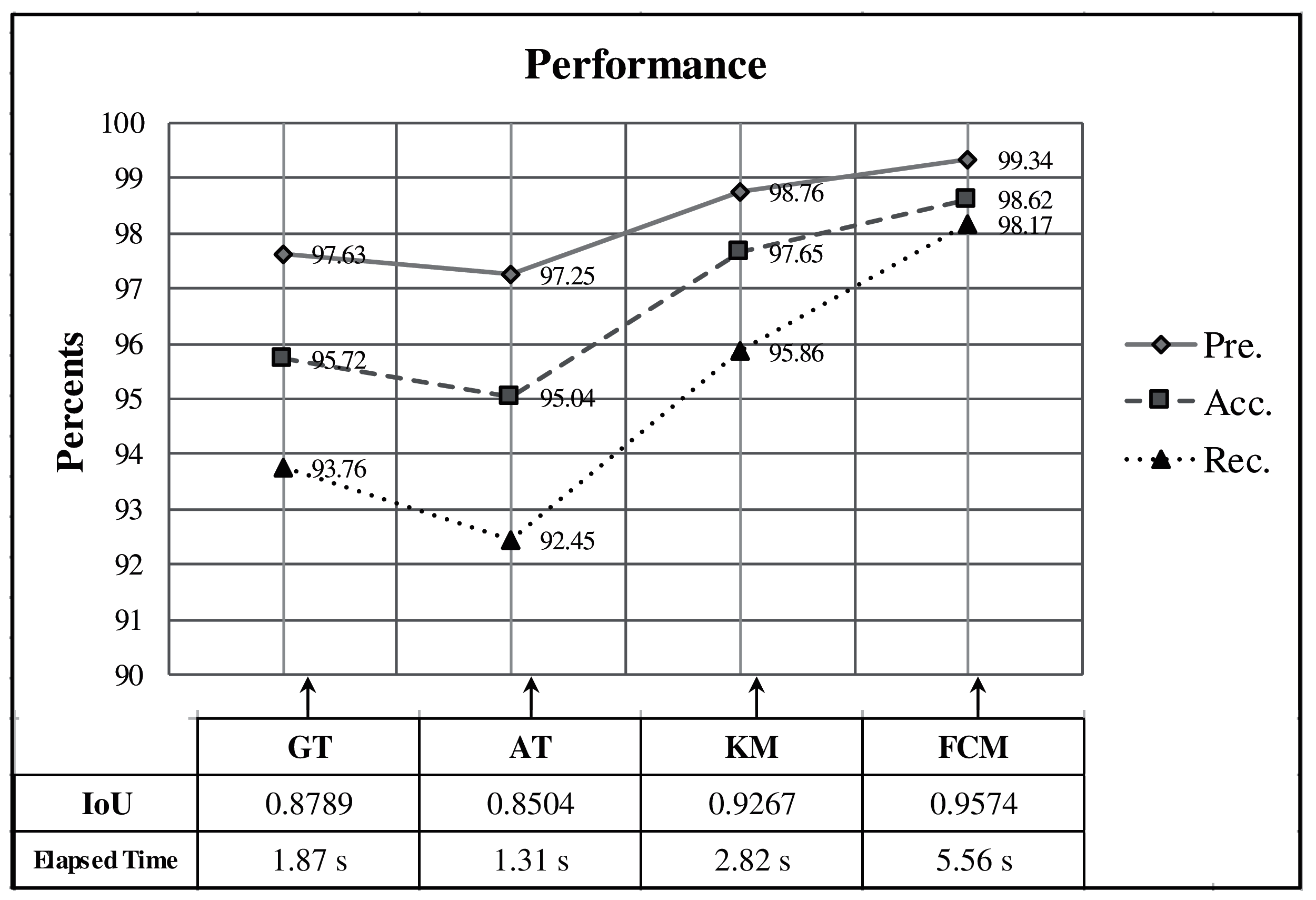Fig.ure 5: The performance of alternative image segmentation algorithms. GT, AT, KM, and FCM respectively denote global thresholding, adaptive thresholding, k-means clustering, and fuzzy c-means clustering. Pre., Acc., and Rec. indicate precision, accuracy, and recall, respectively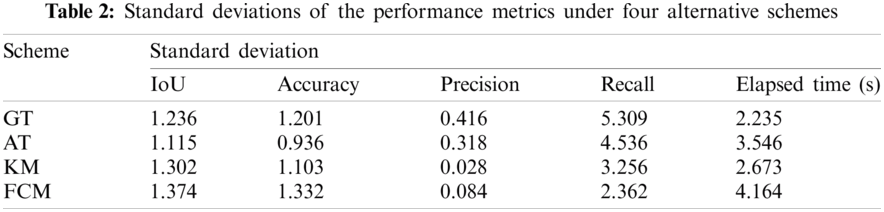Figure 6: Defect detection using image subtraction with white-colored marks indicating detected defects: (a) Defects with a positive subtraction value; (b) Defects with a negative subtraction value; (c) All defects

Defect Classification Performance

CHT, MR, and CCL algorithms are utilized to classify PCB defects according to the 14-known PCB defect types (D01-D14). As discussed above, the defect classification is proposed sequentially and terminated when all 14-known defects are algorithmically classified. The experimental results show that the algorithmic scheme can achieve about 99.05% defect classification accuracy.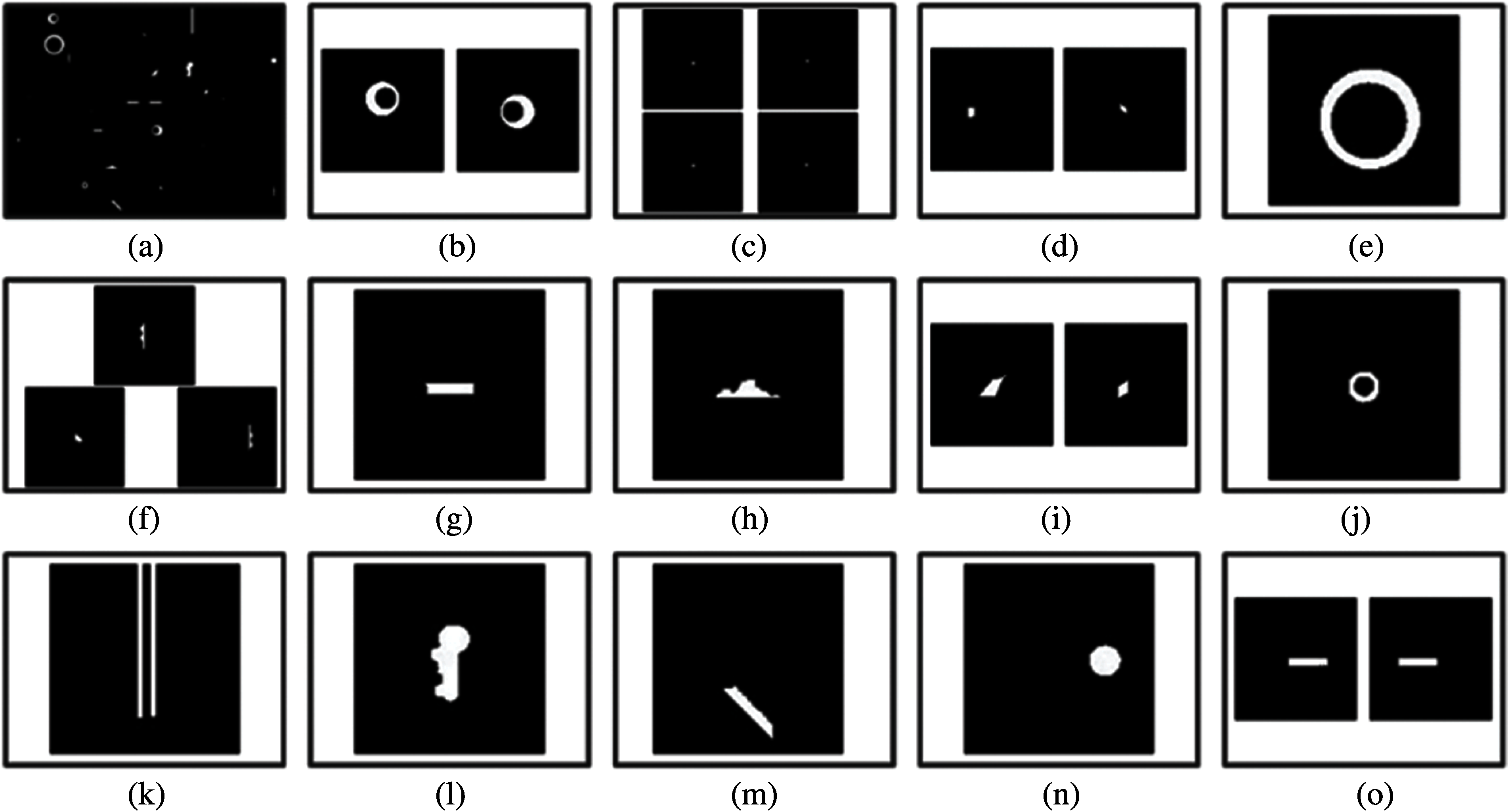Figure 7: The 14-known PCB defect types classified by the proposed algorithmic scheme

Fig. 7a illustrates the 14-known PCB defect types, and Figs. 7b7o illustrate Breakout (D01), Pin hole (D02), Open circuit (D03), Under etch (D04), Mouse bite (D05), Missing conductor (D06), Spur (D07), Short circuit (D08), Wrong size hole (D09), Conductor too close (D10), Spurious copper (D11), Excessive short (D12), Missing hole (D13), and Over etch (D14), respectively.

This research validates the proposed algorithmic scheme by comparing against previously developed methods that including the well-known conventional operations [57,9], the convolutional neural networks [11,12], and the k-nearest neighbor method . In Tab. 3, one finds that in [57,9,11,12] can identify only some types of defects, while  and the proposed algorithm can detect all 14 defect types. Considering the accuracy perspective, it is clear that the accuracy rate of  is about 70%−80%, while our accuracy rate is 96.5%−100%.

As the proposed method resides at the nexus of the CHT, MR and CCL algorithms, the features of these algorithms allow the performance of the proposed algorithmic scheme to detect defects more comprehensively and more accurately than other methods, as shown in Tab. 3.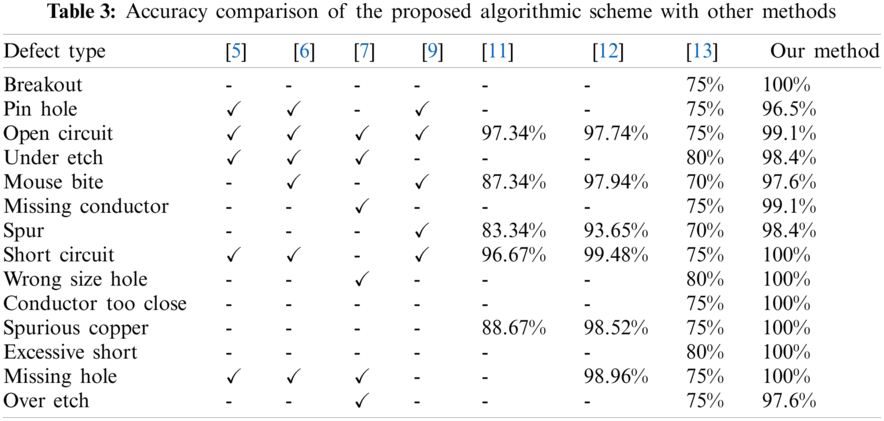4  Conclusion

This study proposes the PCB defect inspection system using a referential approach. The novelty of this work lies in the concurrent use of arithmetic and logical operations, as well as CHT, MR, and CCL algorithms. This algorithm effectively classifies all 14-known PCB defect types, unlike conventional techniques that can only classify a few kinds of defects or cannot achieve high efficiency. The experimental results reveal that the proposed algorithmic scheme can achieve 100% defect detection efficiency and 99.05% defect classification accuracy, demonstrating that our proposed algorithmic scheme has superior performance to other existing PCB defect classification methods. Given the impressive detection and classification performance, the algorithmic scheme can be integrated into machine vision to streamline the production, improve PCB quality, and lower the production cost as human inspectors will no longer be required for defect classification.

The PCB architecture continues to develop and acquires more complicated structures. The currently designed algorithm may also be required to catch up with the growth of PCB developments. Hence, future work will be focused on detecting and classifying defects occurring during PCB production that exhibits more complex structures and processes.

Acknowledgement: The authors would like to express sincere gratitude to the Signal Processing Research Laboratory, the Faculty of Engineering, Rajamangala University of Technology Thanyaburi, for insight and expertise. Appreciation also goes to Dr. Kan Kanjana of KB Systems (Thailand) Co., Ltd., for valuable comments and suggestions. We also thank TopEdit (https://www.topeditsci.com) for its linguistic assistance during the preparation of this manuscript.

Funding Statement: This research is supported by the National Research Council of Thailand (NRCT). Project ID: 618211.

Conflicts of Interest: The authors declare that they have no conflicts of interest to report regarding the present study.

References

1.  M. Moganti, F. Ercal, C. H. Dagli and S. U. Tsunekawa, “Automatic PCB inspection algorithms: A survey,” Computer Vision and Image Understanding, vol. 63, no. 2, pp. 287–313, 1996.

2.  K. Kaur and B. Kaur, “PCB defect detection and classification using image processing,” International Journal of Emerging Research in Management & Technology, vol. 3, no. 8, pp. 42–46, 2014.

3.  H. Rau, C. Wu, W. Shiang and P. Huang, “A decision support system of statistical process control for printed circuit boards manufacturing,” in 2010 Int. Conf. on Machine Learning and Cybernetics, Qingdao, China, vol. 5, pp. 2454–2458, 2010.

4.  W. Wu, M. J. Wang and C. Liu, “Automated inspection of printed circuit boards through machine vision,” Computers in Industry, vol. 28, no. 2, pp. 103–111, 1996.

5.  A. P. S. Chauhan and S. C. Bhardwaj, “Detection of bare PCB defects by image subtraction method using machine vision,” in Proc. of the World Congress on Engineering 2011, London, U.K., vol. 2, pp. 1597–1601, 2011.

6.  I. Ibrahim, Z. Ibrahim, K. Khalil, M. M. Mokji and S. A. B. S. A. Rahman, “An algorithm for classification of five types of defects on bare printed circuit board,” International Journal of Computer Sciences and Engineering Systems, vol. 5, no. 3, pp. 201–208, 2011.

7.  I. Ibrahim, Z. Ibrahim, K. Khalil, M. M. Mokji, S. A. B. S. A. Rahman et al. “An improved defect classification algorithm for six printing defects and its implementation on real printed circuit board images,” International Journal of Innovative Computing, Information and Control, vol. 8, no. 5A, pp. 3239–3250, 2012.

8.  B. Kaur, G. Kaur and A. Kaur, “Detection and classification of printed circuit board defects using image subtraction method,” in 2014 Recent Advances in Engineering and Computational Sciences (RAECSChandigarh, India, pp. 1–5, 2014.

9.  S. Vishwakarma, S. Tikke, C. Manurkar and A. Thanekar, “PCB detection and classification using digital image processing,” International Journal of Advance Research in Science and Engineering, vol. 4, no. 1, pp. 1486–1490, 2015.

10. A. Raj and A. Sajeena, “Defects detection in PCB using image processing for industrial applications,” in 2018 Second Int. Conf. on Inventive Communication and Computational Technologies (ICICCTChandigarh, India, pp. 1077–1079, 2018.

11. J. Zhu, A. Wu and X. Liu, “Printed circuit board defect visual detection based on wavelet denoising,” IOP Conference Series: Materials Science and Engineering, vol. 392, no. 6, pp. 1–6, 2018.

12. P. Wei, C. Liu, M. Liu, Y. Gao and H. Liu, “CNN-Based reference comparison method for classifying bare PCB defects,” The Journal of Engineering, vol. 2018, no. 16, pp. 1528–1533, 2018.

13. L. Zhang, Y. Jin, X. Yang, X. Li, X. Duan et al. “Convolutional neural network-based multi-label classification of PCB defects,” The Journal of Engineering, vol. 2018, no. 16, pp. 1612–1616, 2018.

14. W. Huang and P. Wei, “A PCB dataset for defects detection and classification,” Journal of LATEX Class Files, vol. 14, no. 8, pp. 1–9, 2018.

15. N. K. Khalid, Z. Ibrahim and M. S. Z. Abidin, “An algorithm to group defects on printed circuit board for automated visual inspection,”International Journal of Simulation: Systems, Science, and Technology, vol. 9, no. 2, pp. 1–10, 2008.

16. S. H. I. Putera and Z. Ibrahim, “Printed circuit board defect detection using mathematical morphology and MATLAB image processing tools,” in 2010 Second Int. Conf. on Education Technology and Computer (ICETCShanghai, China, vol. 5, pp. 359–363, 2010.

17. P. S. Malge and R. S. Nadaf, “PCB defect detection, classification and localization using mathematical morphology and image processing tools,” International Journal of Computer Applications, vol. 87, no. 9, pp. 40–45, 2014.

18. M. H. Thigale, S. Gaikwad, P. Nangare and N. Hule, “Defect detection and classification of printed circuit board using MATLAB,” International Research Journal of Engineering and Technology (IRJET), vol. 4, no. 2, pp. 1812–1816, 2017.

19. S. H. I. Putera, S. F. Dzafaruddin and M. Mohamad, “MATLAB based defect detection and classification of printed circuit board,” in 2012 Second Int. Conf. on Digital Information and Communication Technology and It's Applications (DICTAPBangkok, Thailand, pp. 115–119, 2012.

20. R. Heriansyah, S. A. R. Al-Attas and M. M. A. Zabidi, “Segmentation of PCB images into simple generic patterns using mathematical morphology and windowing technique,” in The National Conf. on Computer Graphic & Multimedia (CoGRAMMMelaka, Malaysia, pp. 233–238, 2002.

21. P. P. Londe and S. A. Chavan, “Automatic PCB defects detection and classification using MATLAB,” International Journal of Current Engineering and Technology, vol. 4, no. 3, pp. 2119–2123, 2014.

22. T. S. Huang, G. J. Yang and G. Y. Tang, “A fast two-dimensional median filtering algorithm,” IEEE transactions on acoustic,” Speech, and Signal Processing, vol. 27, no. 1, pp. 13–18, 1979.

23. I. Ibrahim, S. A. R. S. A. Bakar, M. M. Mokji, J. A. A. Mukred, Z. M. Yusof et al. “A printed circuit board inspection system with defect classification capability,” International Journal of Innovative Management, Information & Production, vol. 3, no. 1, pp. 82–87, 2012.

24. W. Wang, C. Wang, X. Cui and A. Wang, “Improving fuzzy C-means clustering based on adaptive weighting,” in 2008 Fifth Int. Conf. on Fuzzy Systems and Knowledge Discovery, Jinan, China, vol. 1, pp. 62–66, 2008.

25. N. Gothankar, C. Kambhamettu and P. Moser, “Circular hough transform assisted CNN based vehicle axle detection and classification,” in 2019 4th Int. Conf. on Intelligent Transportation Engineering (ICITESingapore, pp. 217–221, 2019.

26. Y. Sharma and Y. K. Meghrajani, “Brain tumor extraction from MRI image using mathematical morphological reconstruction,” in 2014 2nd Int. Conf. on Emerging Technology Trends in Electronics, Communication and Networking, Surat, India, pp. 1–4, 2014.

27. R. Akmal, Z. S. O. Nur, B. Abdullah, S. M. R. Mohd and M. A. Ismail, “Connected component labelling using components neighbours-scan labelling approach,” Journal of Computer Science, vol. 6, no. 10, pp. 1070–1078, 2010.

28. L. Zhao, S. Zou, Y. Zhang, M. Huang, Y. Zuo et al., “Segmentation of activated sludge phase contrast microscopy images using U-net deep learning model,” Sensors and Materials, vol. 31, no. 6, pp. 2013–2028, 2019.This work is licensed under a Creative Commons Attribution 4.0 International License, which permits unrestricted use, distribution, and reproduction in any medium, provided the original work is properly cited.